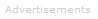# Definition of Gas

Gases are one of the four common states of matter - the others are solids, liquids, gases, and plasmas. In general, but not in all specific cases, as the temperature increases and pressure decreases substances pass through the four different states.

solid → liquid → gas → plasma

## The Gas State

The gaseous state of matter is characterized by the following properties:

• its volume is not fixed; it will expand to fill a container - unlike solids and liquids
• it is compressible - its volume changes when pressure changes - unlike solids and liquids
• gases are fluids, so they can flow. Their shapes adapt to that of their containers
• the atoms or molecules in a gas are spread much more thinly than the particles in solids and liquids. For example, at a temperature of 0 °C and pressure of 1 atm, 1 mole of water has a volume of 18 ml in the liquid state, and 22.4 liters in the vapor state. (At 0 °C and 1 atm, a small amount of vapor exists in equilibrium with liquid/solid water.)

## The Gaseous Elements

At room temperature and atmospheric pressure, eleven of the chemical elements exist as gases.

### Noble Gases

Six of these are noble gases, existing as single atoms: helium, neon, argon, krypton, xenon, and radon.

2
He
4.00
10
Ne
20.18
18
Ar
39.95
36
Kr
83.80
54
Xe
131.30
86
Rn
(222)

### Diatomic Gases

Five exist as diatomic molecules: H2, N2, O2, F2, and Cl2.

1
H
1.008
7
N
14.01
8
O
16.00
9
F
19.00
17
Cl
35.45

### Air

Air is a mixture of gases. Three elements make up over 99.9 percent of the composition of dry air: these are N2, O2, and Ar.

7
N
14.01
8
O
16.00
18
Ar
39.95

### The Gas Laws

Ideal Gases
The relationship between the number of moles of a gas and its pressure, volume and temperature can be calculated using the ideal gas law:

PV = nRT
P is pressure in pascals
V is volume in m3
n is number of moles
R is the ideal gas constant = J K-1 mol-1
T is temperature in K

The equation can also be used with other units.

Real Gases
The ideal gas law is based on assumptions that gas particles have no volume and do not attract or repel one another. It is most accurate for gases at low pressures. At higher pressures, other equations can be used, such as the van der Waals equation:

(P + an2/V2) (V - nb) = nRT

a and b are constants that vary depending on the gas.Search the Dictionary for More Terms